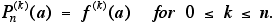MTH 152 Lab 8 03-08-05

Purpose. The purpose of this lab is to motivate studying series and sequences. The basic idea is simple: we try to approximate arbitrary functions, like exponential and trig functions, by polynomials. How do we go about this? Here is a brief summary. We start with a function f and a point a in the domain of f. We then build a polynomial Pn of degree n, called the Taylor polynomial of degree n, using the rule that the first n derivatives of f and Pn should have the same value at a. In other words, the defining principle for Pn is:(Here, we interpret the 0th derivative as just the function itself.) It is possible to write out an explicit formula for Pn, namely:The lab focuses on gaining an intuitive understanding of the relationship between functions and their Taylor polynomials by looking at the graphs of f and of Pn on the same set of axes. You’ll type in a short Maple procedure that allows you to enter f, n, and a and to specify the width and height of the graphs produced. The procedure then shows you what Pn is and displays the graphs of f and Pn on the same set of axes. By applying this procedure for different values of f, n, and a, you should gain an appreciation for how Taylor polynomials approximate functions.

Instructions
. First type:

taygraph:=proc(f,n,c,a,b) local i,s;
s:=eval(subs(x=c,f));
for i from 1 to n do
s:=s+eval(subs(x=c,diff(f,x\$i)))/i!*(x-c)^i
od;
print(f,s);
plot([f,s],x=c-a..c+a,-b..b);
end;

1. Explore the approximations to sin(x) at a = 0. Begin with n=1, width = Pi, and height = 1.5. Note that the format for taygraph is taygraph(function,a,n,width, height). Try increasing the value of n (by 1 each time) until the graph of the Taylor polynomial and the sine function are indistinguishable on the graph. At this point, triple the width of the interval (to 3*Pi). What do you see? Now try increasing n again until the two graphs nearly coincide. Then expand the interval once again to a width of 10*Pi. How large does n have to be for the two graphs to be nearly the same on this new interval? Do you think that there is any end to how wide the interval can be if we are willing to make n large enough?

2. Repeat question 1 with a = 3Pi/4. What similarities do you see? What differences do you see?

3. Explore the Taylor polynomial approximations to the exponential function exp(x). For this function, you will have to adjust the value of height as you adjust the width so that you see the whole graph. Start with a = 0, n = 1, width = 2, and height = 8. Proceed as in question 1. Do you think that there is any end to how wide the interval can be?